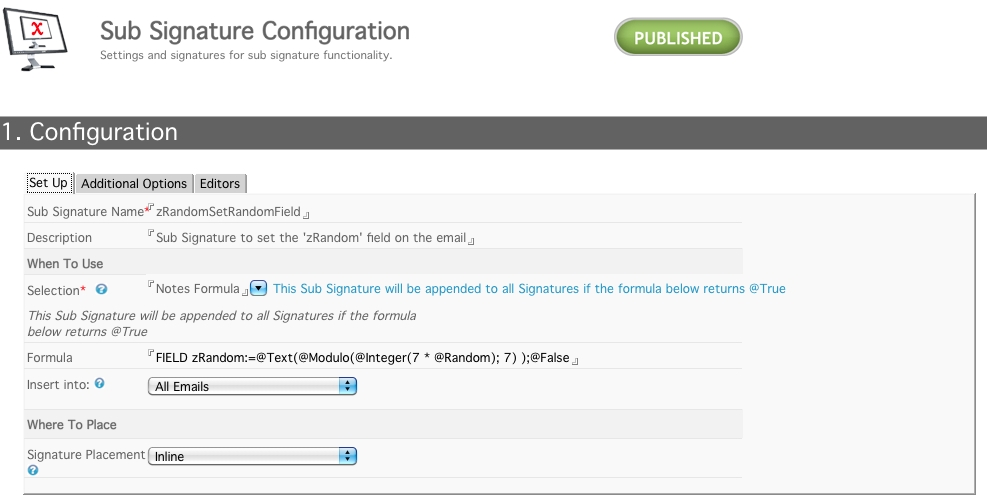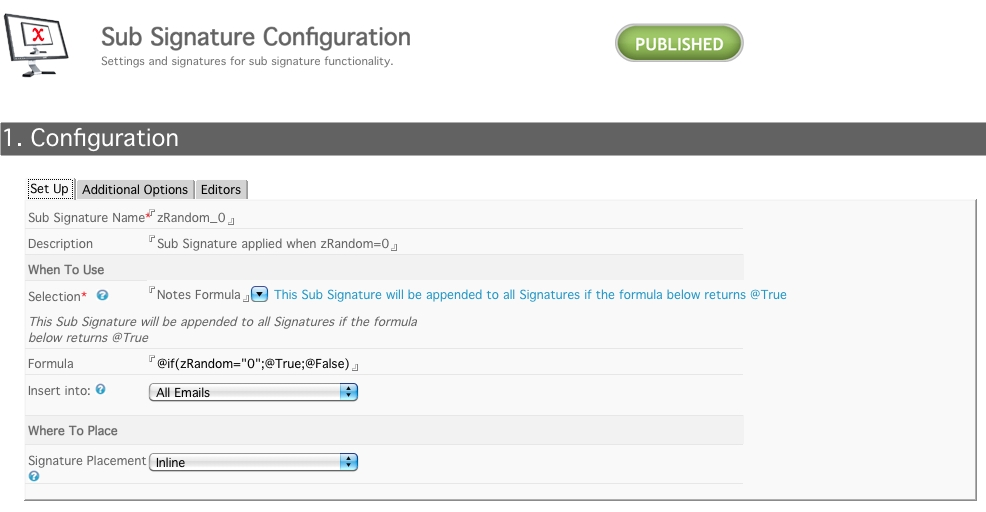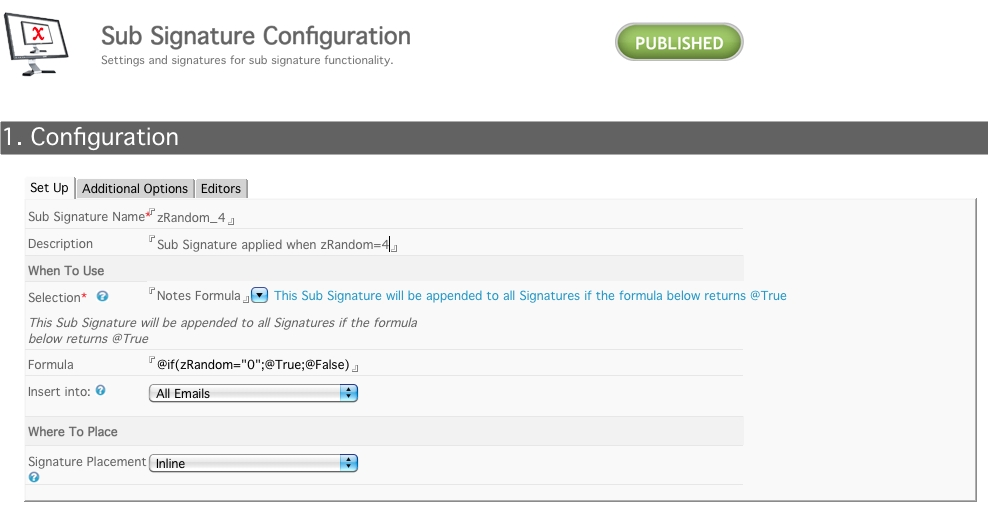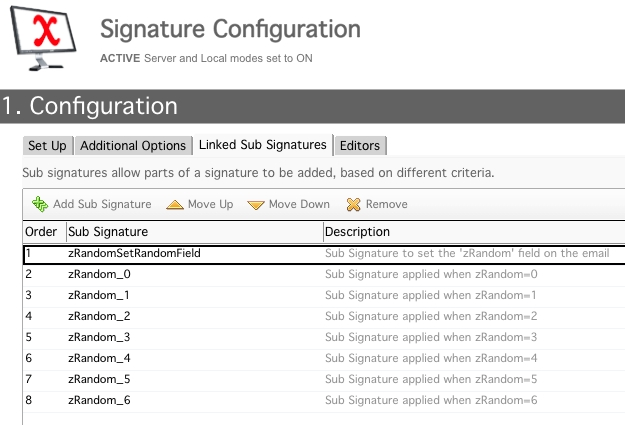Views:

This example of a random sub signature is based on generating a random number in the first sub signature using a formula.

This example allows for easier control on the sub signature formulas compared to the example based on time: Example Sub signatures

### Create a sub signature that generates a random number

This sub signature NEVER gets applied. The notes formula always returns false, but part of the formula writes the random number to the email.

This sub signature MUST always be the first to be evaluated.

When the next sub signature formula is evaluated it can check the field in the email and compare it the value it its formula.`FIELD zRandom:=@Text(@Modulo(@Integer(7 * @Random); 7) );@False`

The above formula generates a random number between 0 and 6. Change the two 7's in the formula to the number of random sub signatures your require.

### Create the random sub signatures

Create a set of sub signatures which contain the Notes Formula below

a. If the zRandom number returns 0b. If zRandom number returns 4### Link to a Signature Configuration

You must then link all the sub signatures to a Signature Configuration document.

The order of the random sub signatures is unimportant, but the sub signature used to generate the random number MUST be the first.Related Products: CMS DOM v4